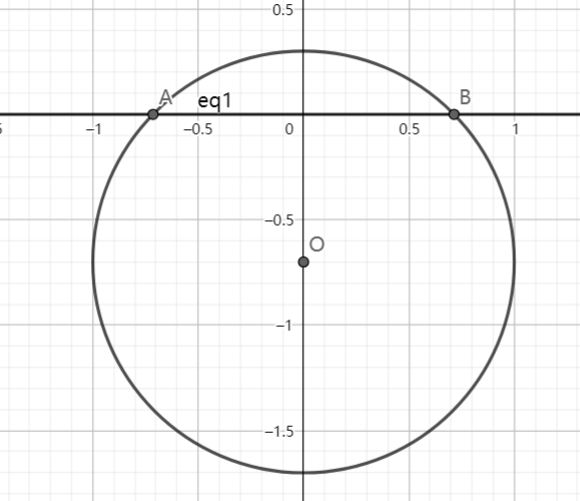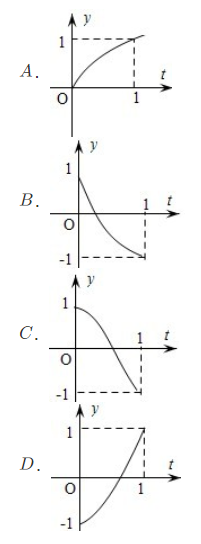# SAT1000 - P329

Geometry Level pendingAs shown above, the circle has radius $r=1\ m$, $O$ is its center. At $t=0$, $O$ is at $(0,-1)$, and it is moving at $v=1\ m/s$ upwards along the y-axis. Let $x(t)$ be the length of the arc above the x-axis, $f(t)=\cos (x(t))$.

For $0 \leq t \leq 1$, what is the best graph for $f(t)$?Have a look at my problem set: SAT 1000 problems

×